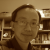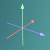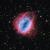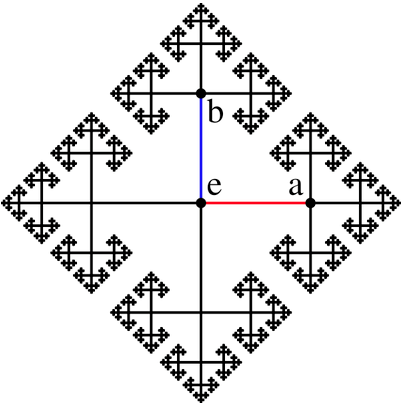# Fractal## Chaos game - square fractal

Chaos game is a mathematical way to generate fractals. It has a simple algorithm, which (1) starts from a radom point with a polygon, then (2) randomly selects a vertice, (3) plots a new point midway between the starting point and the selected vertice. The ploted mid point then becomes the step (1) and reiterates the steps as many times as you like to generate a geometric figure. The example below in this blog uses a square as the polygon to generate a chaos game square fractal.## Dragon curve 2## Geometry exploration

This collection of shapes is the result of my learning the LOGO programming language.## Cayley graph 3DI was in the keynote session of AAMT conference. In the keynote, the mathematician Hanna Neumann was mentioned. I immediately googled and started reading about her on Wikipedia.

While reading, my thoughts are like the hyperlinks that go everywhere, then suddenly I saw and clicked into the Group theory, where I found the Cayley graph that caught my attention.

It is easily recognisable that this cayley graph is a fractal image, which can be produced with a simple recursive procedure in VRMath2's LOGO language.## Recursive 2D fern leaf procedure

In my previous blog, I explained about a recursive tree procedure. Here is another one borrowed from Joshua's Logo Interpreter example: fern leaf procedure. This fern leaf procedure creates a 2D fern leaf in VRMath2's 3D space. You can examine it below in the 3D space with the LOGO program.## Recursive 2D tree

One of the powerful abilities of LOGO programming language is its recursive execution of procedure. Recursive call of procedure means a procedure calls itself until an exiting condition is met. A recursive procedure can be very simple yet it can produce complex and beautiful mathematical graphics such as fractal images and naturally occurring objects.